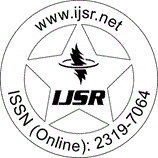International Journal of Science and Research (IJSR)
Call for Papers | Fully Refereed | Open Access | Double Blind Peer Reviewed

Research Paper | Mathematics | India | Volume 2 Issue 9, September 2013

# Implementation of 1D NN in Signal Processing Application to Get Relevant Recurrence Coefficient Value in Discrete Orthogonal Polynomial

N. V. Narendra Babu | G. Manoj Someswar 

Abstract: In the implementation of algorithm we are evaluating the indexes of 1-D NN to the alpha by which we will get the relevant recurrence coefficient value in discrete orthogonal polynomial. In mathematics, a recurrence coefficient is an equation that recursively defines a sequence, once one or more initial terms are given and each further term of the sequence is defined as a function of the preceding terms.

Keywords: recurrence coefficient, difference equation, linear recurrence sequence, infinite impulse response, PSNR

Edition: Volume 2 Issue 9, September 2013,

Pages: 198 - 204